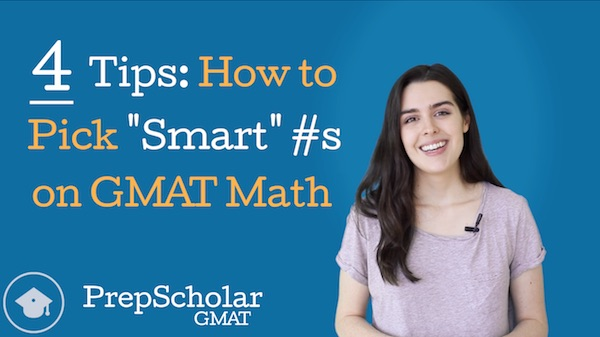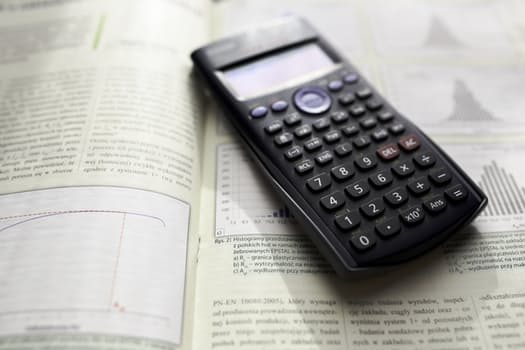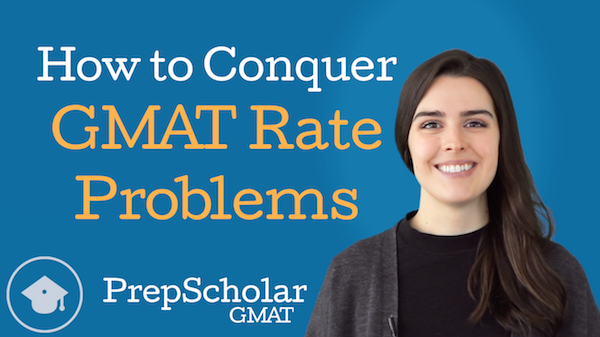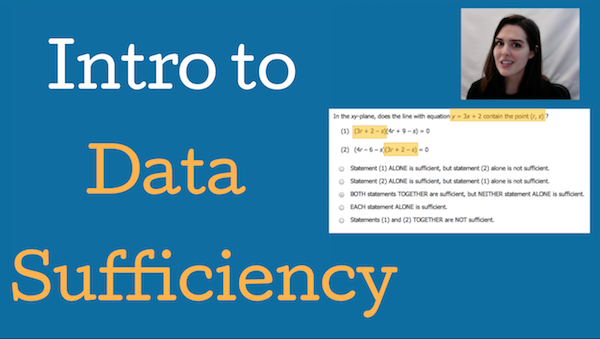## GMAT Remainder Problems: 3 Key TipsIf you’re like me, you probably haven’t thought about remainders in over ten years, when you first learned about long division in elementary school. Remember those lessons on what we call the number that’s left over in a division problem? Yeah, that number’s called a remainder.

And guess what? Remainders show up a lot on the GMAT. Of course, the remainder problems you’ll encounter on the GMAT are much different than the ones you worked on when you were ten years old. GMAT remainder questions can often be quite tricky, but don’t worry! In this guide, I’ll give you a comprehensive overview of GMAT remainder problems so that you feel ready to solve them when you see them on test day.

## How to Plug in “Smart” Numbers on GMAT Math: 4 Tips [Video]Like we discussed in our Online Lesson: Introduction to Data Sufficiency video, picking numbers should not be our go-to strategy for most questions on the GMAT Math section. Most problem types aren’t easily solved by plugging in numbers. Then, even on the problems where number picking is a viable strategy, most test takers approach it incorrectly, which causes them to waste time and confuse themselves. So when is this strategy actually useful? And how can we use it to improve our GMAT Math score?

In “How to Plug in ‘Smart’ Numbers on GMAT Math Problems: 4 Tips”, we’ll teach you how apply one of the most misused GMAT Math strategies successfully on test day with four GMAT Math tips.

While number picking shouldn’t be our first resort on any GMAT Math problem, some questions lend themselves more to number picking than others. Our first tip explores how we can identify questions that are good candidates for number picking.

In our second tip, we delve into our goals for number picking. One of the reasons that number picking is not always an effective strategy is that test takers often plug numbers in randomly without considering how the result will impact which answer choice they’ll pick. We look at how we should have different objectives for different GMAT Math question types, and how these goals will impact the types of numbers (as well as how many numbers) we pick.

Our third and fourth GMAT Math tips explore how to pick “smart” numbers to meet these goals. First, we look at how thinking about categories of numbers can give us a window into which numbers “work” in our problem and which numbers don’t. Then, we consider how to pick specific numbers within these categories for quick math without a calculator.

To stay updated on our latest GMAT videos, you can subscribe to our new PrepScholar GMAT YouTube channel — we’ll have three new free videos every month.

Happy GMAT Math studies!

## The Best GMAT Math Practice: 500+ Questions and TestsThe quantitative section is probably the most notorious and daunting section of the GMAT exam. It can feel like you need to be a genius to get a good score, but really all you need is practice!

In this guide, I’ll explain what you need to prepare for the GMAT quant section and list the best resources for GMAT math practice. Last but not least, you’ll see my best study tips for the math section to help you achieve your goal score.

## GMAT Quantitative: 10 Tips to Master the Math SectionThe GMAT quantitative section is probably the most notorious and daunting section of the exam. There are many myths surrounding the quant section of the GMAT, such as that it tests extremely advanced math concepts or that it’s impossible to achieve a perfect score. However, by building your understanding of the quant section through careful preparation, it’s more than possible to do well on this challenging part of the GMAT.

In this complete GMAT quantitative review, I’ll be giving you an in-depth look into the format of the section and what skills are tested on it. I’ll also take you through a detailed look at the two types of GMAT quant questions (data sufficiency and problem solving). Finally, I’ll give you tips that’ll help you achieve success in your test preparation and on test day.

## How to Conquer GMAT Rate Problems [Video]GMAT rate problems are a constant on the Quantitative section. Many students dread rate problems because they aren’t quick or easy — they often require a series of steps to get to the answer. To make matters worse, this series of steps is usually not immediately obvious because there are so many elements to the problem and because these elements are usually presented as part of a difficult-to-interpret word problem scenario. This means that seeing a clear path to the finish line is difficult with many GMAT rate problems.

So what should we do when we run into a rate problem that we just can’t see how to solve? In “How to Conquer GMAT Rate Problems”, we discuss one of the most valuable GMAT math strategies — taking complex problems step-by-step.

GMAT rate problems give us limited information that we can use in limited ways. At any given step, we are only able to do one or two things with the information we have. Because our options at each step are so limited, we can usually make our way to the answer by figuring out only the next step we are able to take now given the information we have, then repeating the process with the information we learn at each step until we reach our answer. While working out a full, multi-step game plan up top can be overwhelming, simply answering “What can I do with this information?” at each step is more manageable and will eventually lead us to our answer.

In the case of GMAT rate problems, the next step we are able to take is usually doing the next possible conversion — converting the value we have into any another value we can using rates from the problem. We walk through exactly what this means by solving one of the real GMAT rate problems from the GMATPrep software.

Watch the video to see how it’s done!

With this strategy, you’re ready for any multi-step rate problems GMAT test writers can throw at you. However, this isn’t all — taking problems one step at a time when the solution isn’t immediately clear is one of the broader GMAT math strategies, as we can apply it to a variety of multi-step problems. Not sure what to do with a bunch of terms with exponents? See how you can combine two or three of them, then see if it simplifies further with your new term. Have a complicated geometric figure? See which angle or side you can solve for now, then see if you can solve for more with the new information.

As a final note, this strategy applies best to distance rate problems, but it can also be used on certain work rate and interest rate questions. If you’d like more detailed information on how to solve each of these specific types of rate problem, check out our rundown of the three types of GMAT rate problems.

To stay updated on our latest GMAT videos, you can subscribe to our new PrepScholar GMAT YouTube channel — we’ll have three new free videos every month.

Happy GMAT studies!

## How to Beat Overlapping Sets GMAT Problems [Video]Overlapping sets make for particularly hard GMAT math questions. While they technically fall into the category of arithmetic — a type of math most people feel comfortable with — the complexity and presentation of overlapping sets problems throw many test takers for a loop.

So how can test takers make sense of all of the complicated information in these questions? And how can we get overlapping sets GMAT problems right every time? In the new video “How to Beat Overlapping Sets GMAT Problems”, we discuss the number one strategy to conquer these deceptively difficult GMAT questions.

We start off by defining overlapping sets GMAT problems so you can recognize them on test day: they are word problems that deal with one large group that is being evaluated for multiple traits. This in essence breaks our larger group into many smaller groups with different combinations of traits.

We then delve onto our key strategy for overlapping sets GMAT problems: making a diagram. This allows us to

1. organize information from the problem.
2. clarify relationships between our smaller groups.
3. build equations we can then use to solve.

On overlapping sets GMAT problems where our larger group is being evaluated for two traits, we use a specific type of diagram called a “double matrix”, which we explain how to create on your scratch paper. We also use a real overlapping sets GMAT question to illustrate how to set up our double matrix diagram and then use it to solve. Finally, we talk about how to set up a diagram for the really hard GMAT math questions in which our overlapping sets involve evaluating for three or more traits.

Watch the video to see how it’s done!

To stay updated on our latest GMAT videos, you can subscribe to our new PrepScholar GMAT YouTube channel — we’ll have three new free videos every month.

Happy GMAT studies!

## Online Lesson: Introduction to GMAT Data Sufficiency [Video]Many students think that Data Sufficiency is one of the hardest question types on the GMAT. Data Sufficiency is unlike anything they’ve ever seen before, either on tests or in their undergraduate math classes. In “Online Lesson: Introduction to GMAT Data Sufficiency”, we cover the basics of GMAT Data Sufficiency to familiarize you with your new favorite GMAT question type and give you strategies you can apply on test day.

Since this Introduction to GMAT video is relatively long, we’ve broken it down into bite-sized sections focused on key Data Sufficiency topics:

In Overview of GMAT Quant, we discuss the makeup of the GMAT Quantitative section, answering the question “How many Data Sufficiency questions are there on the GMAT?” In Structure of Data Sufficiency questions, we review the three components of a Data Sufficiency problem (the question stem, the statements, and the answer choices) and discuss Data Sufficiency GMAT tips related to this structure. We then delve into the answer choices with What is Sufficiency?”by discussing what the “sufficiency” in GMAT Data Sufficiency means and looking at the two different kinds of sufficiency we see in Data Sufficiency questions.

Once we’ve covered the foundations of GMAT Data Sufficiency, we take a detour for What’s the Point of Data Sufficiency? to discuss how Data Sufficiency problems test skills critical to success in business school and careers. We then discuss How to Approach Data Sufficiency Questions at a broad level, walking chronologically through the steps test takers can apply on every Data Sufficiency problem and providing additional GMAT Data Sufficiency tips. Finally, we walk through more specific Data Sufficiency GMAT Strategy in Data Sufficiency Strategies, covering four of the most useful strategies for GMAT Data Sufficiency.

To stay updated on our latest GMAT videos, you can subscribe to our new PrepScholar GMAT YouTube channel — we’ll have three new free videos every month.

Happy GMAT Data Sufficiency studies!

## What Math Is on the GMAT? Topics, Questions, and ReviewThe Quantitative section of the GMAT strikes fear into the hearts of many test takers who haven’t taken a math class in years. If you’re one of those test takers, you shouldn’t worry! You can totally handle the math in this section.

While the Quantitative section is challenging, it doesn’t test especially advanced concepts. This guide will show you what math is on the GMAT, along with key tips on how to prepare.

First, let’s discuss a general overview of GMAT math. Continue reading “What Math Is on the GMAT? Topics, Questions, and Review”

## GMAT Calculator: What Is It? When Can You Use It?Can you use a calculator on the GMAT? Yes, but probably not as much as you’d like.

In this article, I’ll ago over which sections of the GMAT have an onscreen calculator, the functions of the GMAT calculator, and how to use it most effectively on test day. Continue reading “GMAT Calculator: What Is It? When Can You Use It?”

## The 5 Hardest GMAT Data Sufficiency QuestionsFor many GMAT test takers, Data Sufficiency questions are the most difficult questions on the GMAT. But what do the hardest GMAT Data Sufficiency questions look like? What skills and concepts do they test? What do they have in common? What Data Sufficiency strategies can we use to get these challenging GMAT Quantitative questions right?

In this article, I’ll go over the five hardest GMAT Data Sufficiency questions, what you’ll need to know to solve them, how to approach them on test day, and what we can learn from hard GMAT Quant questions about mastering Data Sufficiency.